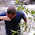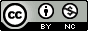## Tuesday, August 28, 2012

### More on Exploring Correlations in R

About a year ago I wrote a post about producing scatterplot matrices in R. These are handy for quickly getting a sense of the correlations that exist in your data. Recently someone asked me to pull out some relevant statistics (correlation coefficient and p-value) into tabular format to publish beside a scatterplot matrix. The built-in cor() function will produce a correlation matrix, but what if you want p-values for those correlation coefficients? Also, instead of a matrix, how might you get these statistics in tabular format (variable i, variable j, r, and p, for each i-j combination)? Here's the code (you'll need the PerformanceAnalytics package to produce the plot).

The cor() function will produce a basic correlation matrix.  12 years ago Bill Venables provided a function on the R help mailing list for replacing the upper triangle of the correlation matrix with the p-values for those correlations (based on the known relationship between t and r). The cor.prob() function will produce this matrix.

Finally, the flattenSquareMatrix() function will "flatten" this matrix to four columns: one column for variable i, one for variable j, one for their correlation, and another for their p-value (thanks to Chris Wallace on StackOverflow for helping out with this one).

Finally, the chart.Correlation() function from the PerformanceAnalytics package produces a very nice scatterplot matrix, with histograms, kernel density overlays, absolute correlations, and significance asterisks (0.05, 0.01, 0.001):

1.This comment has been removed by the author.

2.Amazing graphic ! I'll put this in my preference list. Thanks for share. :)

3.Thanks for the post. chart.Correlation is very useful. Here's a little piece I wrote about using the correlation dimension to get a feeling for the distortions caused by groups of highly correlated variables, assuming one is looking for (groups of high) correlations as something to eliminate.

4.Thanks for sharing Stephen! Your blog is always a solid read.

5.Was there any particular reason for reinventing cor.test()?

6.cor() gives you correlations for all pairwise numeric vectors, and the cor.prob() function above extends this to give you both the correlation and cor.test() for pairwise combination.

7.Hey, Stephen! This is amazing! I'm a total statistics noob, and I'm confused about what information the plots in the lower half of the circle are actually giving. Any help?

1.The plots below the diagonal are just scatterplots. E.g., if you want to see how dispersion and weight relate, follow the "wt" to the left, and "disp" down, and where they intersect is the scatterplot (with a lowess smooth overlay). E.g. circled in blue in this image.

2.*facePalm*. Of course. Thank you.

8.Hey, Stephen!

After working a little more with the chart.correlation function, I've got a number of issues that I've encountered:

1. I'm working with a somewhat large matrix of traits, such that when the chart is generated, each cell is super small. Is there any way to increase the absolute size of the chart, so that the data are actually visible?

2. Similarly, some of my traits have long-ish names, and I was wondering whether there would be a way to wrap the text in the histogram cells (I could change the names of the traits, of course)...

3. Finally, I'm noticing that my correlation chart is not a symmetrical matrix in the end (there are several extra columns that don't respond to any additional rows, and it's unclear what trait correlation coefficients are being displayed in them). I'm wondering whether this has something to do with missing data in my dataset (I got several "the standard deviation is zero" warnings, and also an "Error in cor.test.default(x, y) : not enough finite observations" message).

Any help or suggestions that you might have would be extremely appreciated! (And I'd be more than happy to send you my data and/or pictures of the chart I have so far privately).

1.1. If you type the name of the function without the parentheses, you'll see the source code in there. Try modifying or passing a parameter like cex.labels=0.5.

2. Best to try the cex.labels argument or else change the variable names.

3. This could be any number of things. Sounds like something's wrong with your data. Try using small subsets of your data to nail down the problem. Look for NA's. Look for zeros. Look for NaN's or Inf's. This is part of why data visualization is important - it immediately tells you when you have issues with your data!

9.Thanks so much, Stephen! Now I've got a place to start! Much appreciated.

10.Great piece of code...thanks!!! Is there a way to show the sign of the correlations instead of absolute values?

11.Hm, you'll probably want to modify the code in the chart.Correlation function in the PerformanceAnalytics package. I didn't write this code.

12.Thank you for the beautiful scatterplot matrix code! For my agricultural data, I would like to replace the lowess overlay line in the lower left scatterplots with a linear regression line and show the slope (m= ) and intercept (y=). is this possible?

1.Probably. Have a look at the source code for the chart.Correlation() function.

2.I just started learning R (on my own) so I am not sure where to find the source code, is it in the "PerformanceAnalytics" package? Do you have a recommendation as to what code to add/replace?

3.Just load the package and type the name of the function and look around for where it's doing the lowess fitting and mod that to use lm instead. Something like that.

library(PerformanceAnalytics)

chart.Correlation

Note: Only a member of this blog may post a comment.